Next: Definition of Chaos Up: The Chaotic Pendulum Previous: Route to Chaos

Sensitivity to Initial Conditions

Suppose that we launch our pendulum and then wait until its motion has converged onto a particular attractor. The subsequent motion can be visualized as a trajectory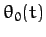,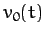through phase-space. Suppose that we then somehow perturb the pendulum, at time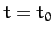, such that its position in phase-space is instantaneously changed from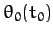,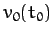to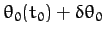,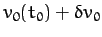. The subsequent motion can be visualized as a second trajectory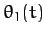,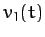through phase-space. What is the relationship between the original trajectory,and the perturbed trajectory,? In particular, does the phase-space separation between the two trajectories, whose components are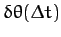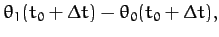(1255)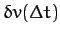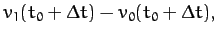(1256)

grow in time, decay in time, or stay more or less the same? What we are really investigating is how sensitive the time-asymptotic motion of the pendulum is to initial conditions.

According to the linear analysis of Section 15.3,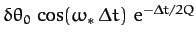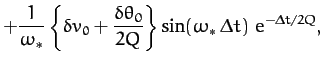(1257)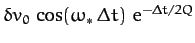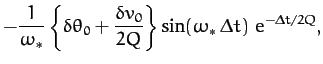(1258)

assuming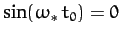. It is clear that, in the linear regime, at least, the pendulum's time-asymptotic motion is not particularly sensitive to initial conditions. In fact, if we move the pendulum's phase-space trajectory slightly off the linear attractor, as described above, then the perturbed trajectory decays back to the attractor exponentially in time. In other words, if we wait long enough then the perturbed and unperturbed motions of the pendulum become effectively indistinguishable. Let us now investigate whether this insensitivity to initial conditions carries over into the non-linear regime.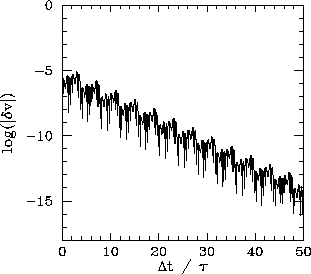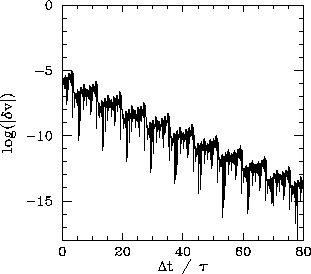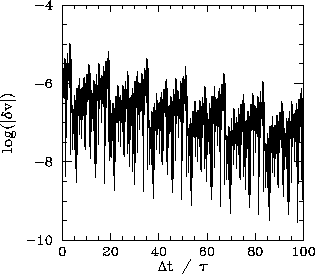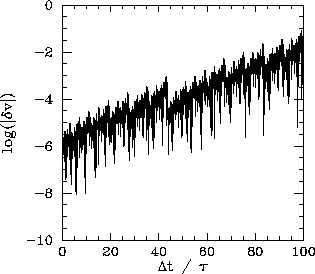Figures 83-86 show the results of the experiment described above, in which the pendulum's phase-space trajectory is moved slightly off an attractor and the phase-space separation between the perturbed and unperturbed trajectories is then monitored as a function of time, at various stages on the period-doubling cascade discussed in the previous section. To be more exact, the figures show the logarithm of the absolute magnitude of the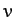-component of the phase-space separation between the perturbed and unperturbed trajectories as a function of normalized time.

Figure 83 shows the time evolution of the-component of the phase-space separation,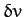, between two neighbouring trajectories, one of which is the period-4 attractor illustrated in Figure 79. It can be seen thatdecays rapidly in time. In fact, the graph of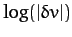versus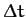can be plausibly represented as a straight-line of gradient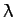. In other words,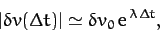(1259)

where the quantityis known as the Liapunov exponent, and is obviously negative. Clearly, in this case,measures the strength of the exponential convergence of the two trajectories in phase-space. Of course, the graph ofversusis not exactly a straight-line. There are deviations due to the fact thatoscillates, as well as decays, in time. There are also deviations because the strength of the exponential convergence between the two trajectories varies along the attractor.

The above definition of the Liapunov exponent is rather inexact, for two main reasons. In the first place, the strength of the exponential convergence/divergence between two neighbouring trajectories in phase-space, one of which is an attractor, generally varies along the attractor. Hence, we should really take formula (1259) and somehow average it over the attractor, in order to obtain a more unambiguous definition of. In the second place, since the dynamical system under investigation is a second-order system, it actually possesses two different Liapunov exponents. Consider the evolution of an infinitesimal circle of perturbed initial conditions, centred on a point in phase-space lying on an attractor. During its evolution, the circle will become distorted into an infinitesimal ellipse. Let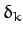, where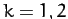, denote the phase-space length of the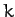th principal axis of the ellipse. The two Liapunov exponents,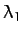and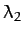, are defined via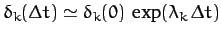. However, for large, the diameter of the ellipse is effectively controlled by the Liapunov exponent with the most positive real part. Hence, when we refer to the Liapunov exponent,, what we generally mean is the Liapunov exponent with the most positive real part.

Figure 84 shows the time evolution of the-component of the phase-space separation,, between two neighbouring trajectories, one of which is the period-8 attractor illustrated in Figure 80. It can be seen thatdecays in time, though not as rapidly as in Figure 83. Another way of saying this is that the Liapunov exponent of the periodic attractor shown in Figure 80 is negative (i.e., it has a negative real part), but not as negative as that of the periodic attractor shown in Figure 79.

Figure 85 shows the time evolution of the-component of the phase-space separation,, between two neighbouring trajectories, one of which is the period-16 attractor illustrated in Figure 81. It can be seen thatdecays weakly in time. In other words, the Liapunov exponent of the periodic attractor shown in Figure 81 is small and negative.

Finally, Figure 86 shows the time evolution of the-component of the phase-space separation,, between two neighbouring trajectories, one of which is the chaotic attractor illustrated in Figure 82. It can be seen thatincreases in time. In other words, the Liapunov exponent of the chaotic attractor shown in Figure 82 is positive. Further investigation reveals that, as the control parameter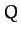is gradually increased, the Liapunov exponent changes sign and becomes positive at exactly the same point that chaos ensues in Figure 78.

The above discussion strongly suggests that periodic attractors are characterized by negative Liapunov exponents, whereas chaotic attractors are characterized by positive exponents. But, how can an attractor have a positive Liapunov exponent? Surely, a positive exponent necessarily implies that neighbouring phase-space trajectories diverge from the attractor (and, hence, that the attractor is not a true attractor)? It turns out that this is not the case. The chaotic attractor shown in Figure 82 is a true attractor, in the sense that neighbouring trajectories rapidly converge onto it--i.e., after a few periods of the external drive their Poincaré sections plot out the same four-line segment shown in Figure 82. Thus, the exponential divergence of neighbouring trajectories, characteristic of chaotic attractors, takes place within the attractor itself. Obviously, this exponential divergence must come to an end when the phase-space separation of the trajectories becomes comparable to the extent of the attractor.

A dynamical system characterized by a positive Liapunov exponent,, has a time horizon beyond which regular deterministic prediction breaks down. Suppose that we measure the initial conditions of an experimental system very accurately. Obviously, no measurement is perfect: there is always some error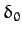between our estimate and the true initial state. After a time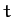, the discrepancy grows to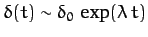. Let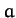be a measure of our tolerance: i.e., a prediction withinof the true state is considered acceptable. It follows that our prediction becomes unacceptable when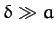, which occurs when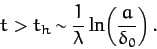(1260)

Note the logarithmic dependence on. This ensures that, in practice, no matter how hard we work to reduce our initial measurement error, we cannot predict the behaviour of the system for longer than a few multiples of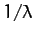.

It follows, from the above discussion, that chaotic attractors are associated with motion which is essentially unpredictable. In other words, if we attempt to integrate the equations of motion of a chaotic system then even the slightest error made in the initial conditions will be amplified exponentially over time, and will rapidly destroy the accuracy of our prediction. Eventually, all that we will be able to say is that the motion lies somewhere on the chaotic attractor in phase-space, but exactly where it lies on the attractor at any given time will be unknown to us.

The hyper-sensitivity of chaotic systems to initial conditions is sometimes called the butterfly effect. The idea is that a butterfly flapping its wings in a South American rain-forest could, in principle, affect the weather in Texas (since the atmosphere exhibits chaotic dynamics). This idea was first publicized by the meteorologist Edward Lorenz, who constructed a very crude model of the convection of the atmosphere when it is heated from below by the ground.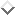Lorenz discovered, much to his surprise, that his model atmosphere exhibited chaotic motion--which, at that time, was virtually unknown. In fact, Lorenz was essentially the first scientist to fully understand the nature and ramifications of chaotic motion in physical systems. In particular, Lorenz realized that the chaotic dynamics of the atmosphere spells the doom of long-term weather forecasting: the best one can hope to achieve is to predict the weather a few days in advance (for the atmosphere is of order a few days).Next: Definition of Chaos Up: The Chaotic Pendulum Previous: Route to Chaos
Richard Fitzpatrick 2011-03-31